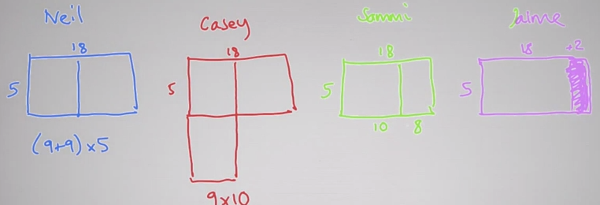^
You are here: HomeLessons → Number talks

# What are number talks?

Number talks (or "math talks") are short discussions among a teacher and students about how to solve a particular mental math problem. The focus is not on the correct answer, but on all the possible methods of finding the answer. Each student has a chance to explain their method, and everyone else will learn from other people's methods!

To start a number talk, the teacher gives the students a SHORT math problem to solve — but the students are not allowed to use a calculator or paper & pencil. The idea is to solve it in one's head! For example, you could ask 4th-12th grade students to solve 5 × 18 using mental math.

(The discussion below is based on Jo Boaler's video on number talks from her website Youcubed.)

The image below shows visual illustrations of FOUR different ways of finding 5 × 18. They are also found in the video below.1. Neil's method was to break 18 into 9 + 9. He calculated 5 × 9 and 5 × 9, and added those.
2. Casey used halving & doubling: he took half of 18 and double of 5, and multiplied those: 9 × 10 = 90.
3. Sammi used the distributive property, breaking 18 into 10 + 8. She multiplied 5 × 10 and 5 × 8 and added those.
4. Jaime solved 5 × 20 = 100, and then subtracted 5 × 2 from that.

As you can see, there are indeed many ways to solve this problem!

Number talks foster number sense — the ability to work with numbers by breaking and decomposing them into parts. And this ability has been found to be of paramount importance for students to be able be proficient in algebra.

Some other problems you can try are 12 × 15 and 21 × 9. Of course you can use this concept with other operations and with fractions and decimals, too. How would you solve 2.75 × 20 or 398 − 199? You can use double-digit additions and subtractions (such as 25 + 26 and 71 − 32) for younger students.

Here's another resource for number talks (they call them "number strings"): Numberstrings.com.

Jo Boaler from Stanford University has made a really good video about the basics of number talks. Please watch it below to learn much more!

I really like the concept of number talks. Students can see that there are MANY ways of seeing the problem and finding the answer. It also helps students to communicate about mathematics — which is a very important skill — and to become more confident in class. In fact, number talks are a great way to help students to overcome a fear of presenting their solutions to others, because the tasks are short and relatively easy (the teacher of course needs to adjust the mental math problem to the students' level).

So please watch the video above.

I have always placed a great emphasis on number sense and mental math in my Math Mammoth books — so much so that many children who come from using other math curricula have difficulties with the mental math questions in the placement tests.

Some of my books with mental math strategies include:

• Add & Subtract 2-B — addition and subtraction of two-digit numbers, including with mental math
• Add & Subtract 3 — addition and subtraction of three-digit numbers including with mental math
• Multiplication 2 — multi-digit multiplication, including mental math problems such as 6 × 20 and 7 × 31
• Decimals 1 — introduction to decimals. You'll learn to solve problems such as 0.05 + 0.2 and 6 × 0.8 using mental math

By Maria Miller

WAIT!

Receive my monthly collection of math tips & resources directly in your inbox — and get a FREE Math Mammoth book!You can unsubscribe at any time.

### Math Mammoth Tour

Confused about the different options? Take a virtual email tour around Math Mammoth! You'll receive:

An initial email to download your GIFT of over 400 free worksheets and sample pages from my books. Six other "TOURSTOP" emails that explain the important things and commonly asked questions concerning Math Mammoth curriculum. (Find out the differences between all these different-colored series!)

This way, you'll have time to digest the information over one or two weeks, plus an opportunity to ask me personally about the curriculum.
A monthly collection of math teaching tips & Math Mammoth updates (unsubscribe any time)### "Mini" Math Teaching Course

This is a little "virtual" 2-week course, where you will receive emails on important topics on teaching math, including:

- How to help a student who is behind
- Troubles with word problems
- Teaching multiplication tables
- Why fractions are so difficult
- The value of mistakes
- Should you use timed tests
- And more!

A GIFT of over 400 free worksheets and sample pages from my books right in the very beginning.A monthly collection of math teaching tips & Math Mammoth updates (unsubscribe any time)
Enter your email to receive math teaching tips, resources, Math Mammoth news & sales, humor, and more! I tend to send out these tips about once monthly, near the beginning of the month, but occasionally you may hear from me twice per month (and sometimes less often).• A GIFT of over 400 free worksheets and sample pages from my books.